##### Search### QUESTION 1

1. 5.01-5 Dijkstra’s Algorithm (1, part 5).  Consider the network shown below, and Dijkstra’s link-state algorithm to find the least cost path from source node U to all other destinations.  Using the algorithm statement and its visual representation used in the textbook, complete the fifth row in the table below showing the link state algorithm’s execution by matching the table entries (a), (b), (c),and (d) with their values.  The *s shown correspond to your earlier answers to this question. [Note: You can find more examples of problems similar to this here.]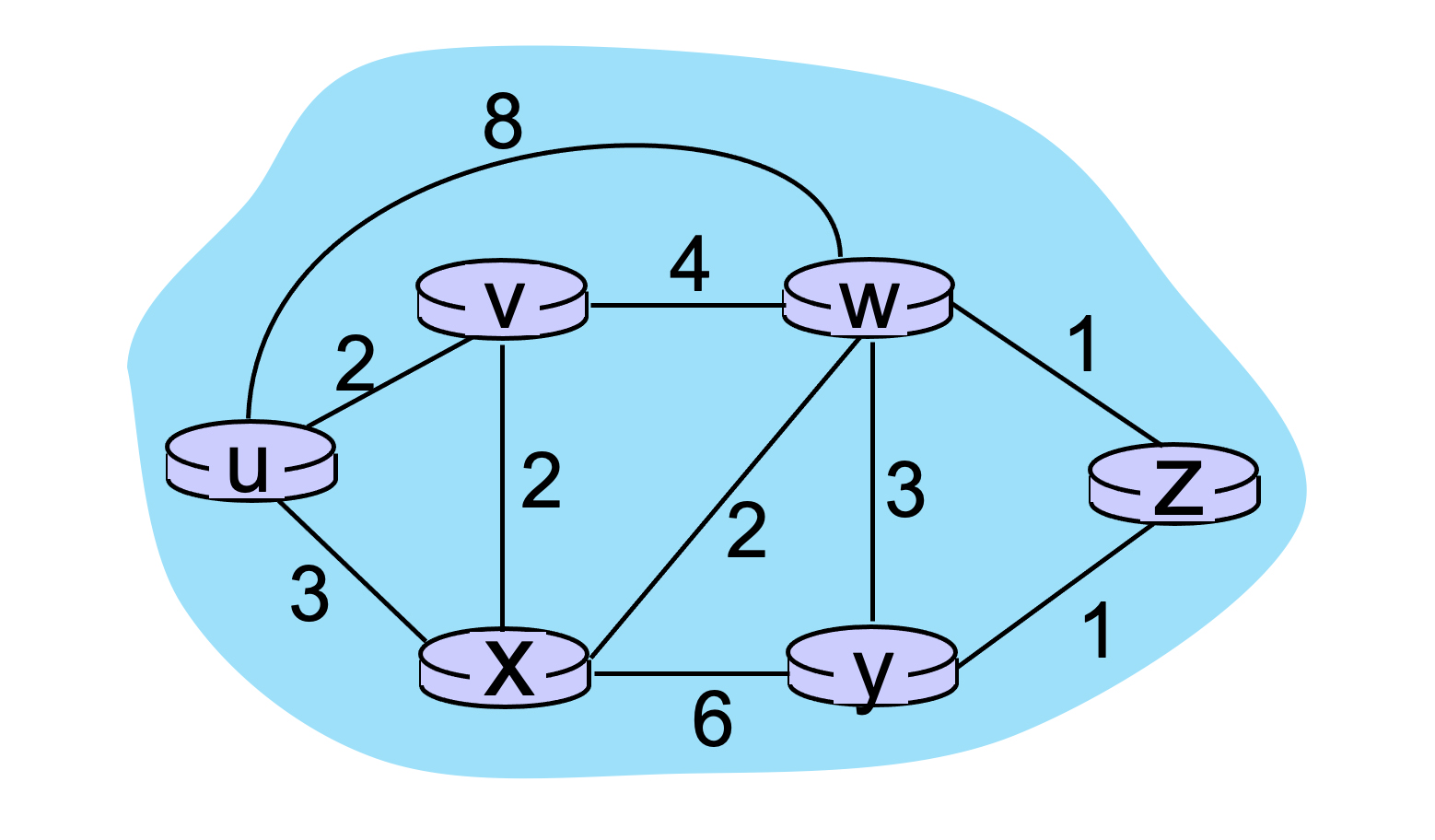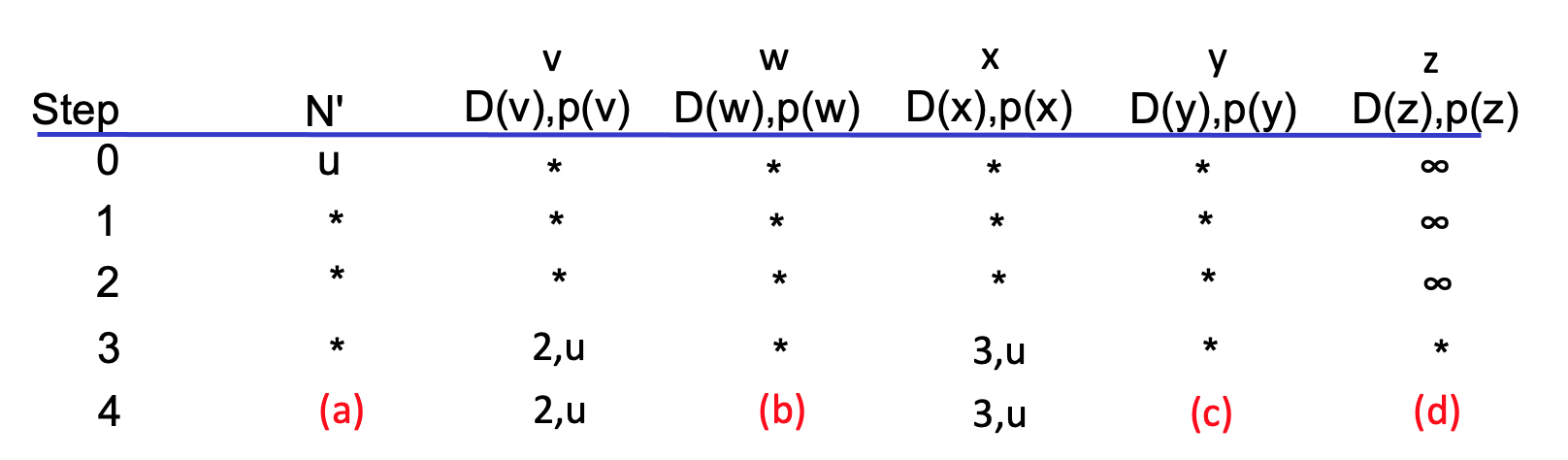–A.B.C.D.E.F.G.H.I. (a) –A.B.C.D.E.F.G.H.I. (b) –A.B.C.D.E.F.G.H.I. (c) –A.B.C.D.E.F.G.H.I. (d)
 A. 5,x B. uvxwz C. uvxwy D. 7,z E. 8,w F. infinity G. 6,v H. 9,x I. 6,w

### QUESTION 2

1. 5.03-3. Dijkstra’s Algorithm (3, part 3).  Consider the network shown below, and Dijkstra’s link-state algorithm. Here, we are interested in computing the least cost path from node E to all other nodes using Dijkstra’s algorithm. Using the algorithm statement used in the textbook and its visual representation, complete the first row in the table below showing the link state algorithm’s execution by matching the table entries (a), (b), (c), (d) and (e) with their values.  Write down your final [correct] answer, as you‘ll need it for the next question. [Note: You can find more examples of problems similar to this here.]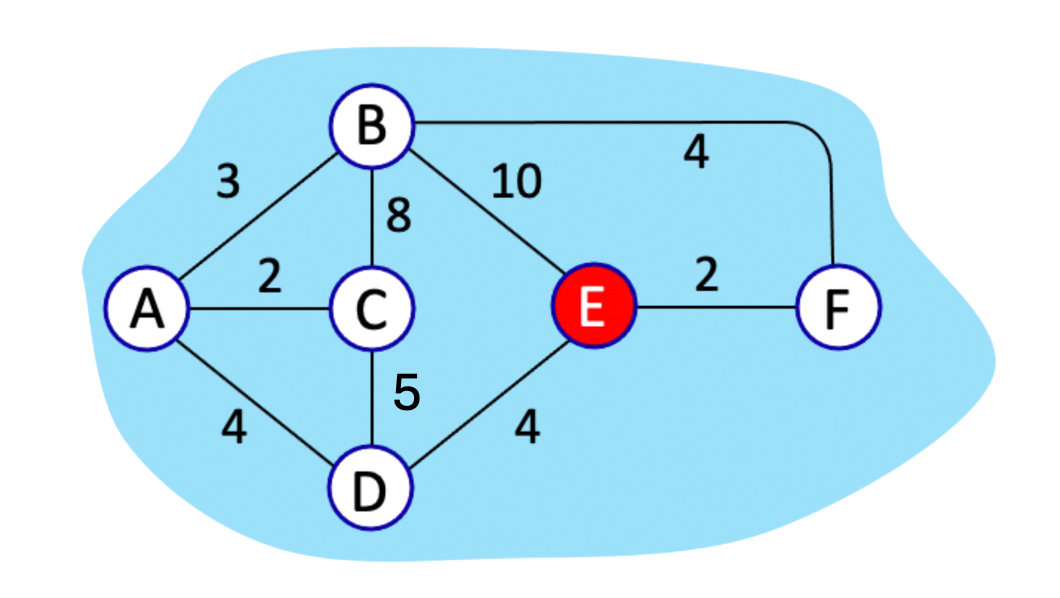–1.2.3.4.5.6.7.8.9.10. (a) –1.2.3.4.5.6.7.8.9.10. (b) –1.2.3.4.5.6.7.8.9.10. (c) –1.2.3.4.5.6.7.8.9.10. (d) –1.2.3.4.5.6.7.8.9.10. (e)
 1 EFB 2 EFD 3 9,D 4 4,E 5 7,D 6 8,F 7 6,D 8 1,E 9 8,D 10 6,F

### QUESTION 3

1. 5.04-4. Bellman Ford Algorithm – a change in DV (1, part 4).  Consider the network below, and suppose that at t=0, the link between nodes b and c goes down. And so at t=0, node b recomputes its distance vector (DV) and sends out its new DV (as needed).  At t=1 this new DV is received at b’s neighbors, who then perform their calculation and send out their new DVs (as needed); these new DVs arrive at their neighbors at t=2, and so on. What is the last time in this network at which a DV calculation will take place as a result of the link change at t=0?3 an essentially infinite amount of time; this is the count-to-infinity problem 4 2

### QUESTION 4

5.05-2. Bellman Ford Algorithm (2, part 2).  Consider the scenario shown below.  The figure below shows the (old) DV at e (before receiving the new DVs from its neighbors) as well as the DVs being sent from b, d, and f.  In the previous quesiton you computed the new DV at ate.  Now suppose that all network nodes have iterated and completed all of the DV calculations, i.e, that the algorithm has converged and quiesced.

Suppose now that sometime after the algorithm has converged, the link between e and f goes down.  Will node e send out a new DV to its neighbors? Pick a response below that best answers this question.No.  Node e’s distance vector does not change when the link between e and f goes down (since e’s shortest path to f did not use this direct link between e and f), so e will not send out a new DV. Yes.  Since node e’s distance vector changes when the link between e and f goes down, it will send out a new DV. Yes.  Node 2 always needs to update all of its neighbors if a alink goes down, since all nodes need to know the network topology.

### QUESTION 5

1. 5.06-3 Bellman Ford Algorithm (3, part 3).  Consider the grid network shown  below. All links have a cost of 1.  Let’s focus on the distance vector (DV) in node L. [Note: You can find more examples of problems similar to this here.]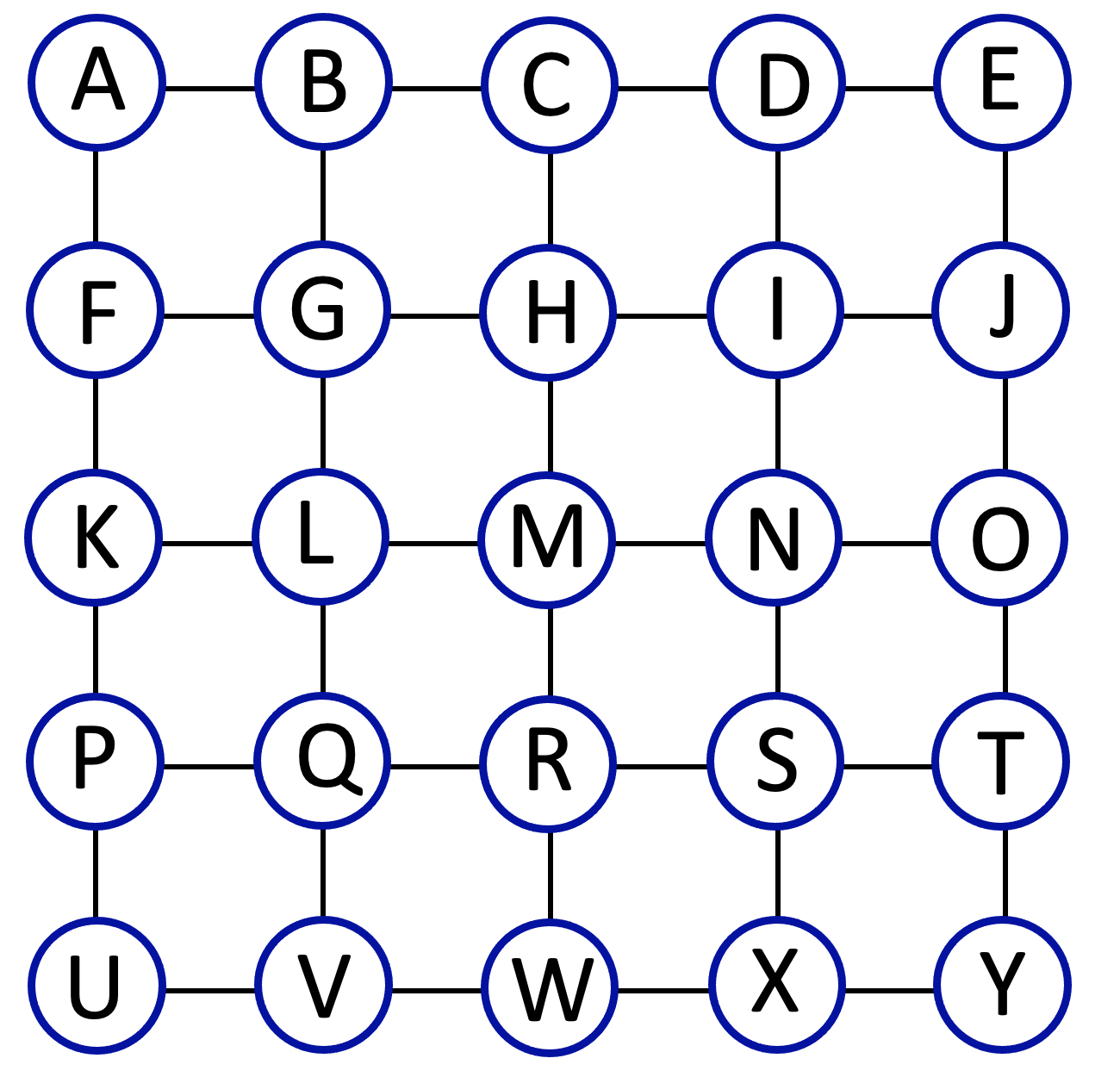Now suppose that the distance vector algorithm has converged.  What are values for the four distance vector elements DL(G), DL(H), DL(I), and DL(E) below in node L? Note: you do not have to run the distance vector algorithm; you should be able to compute distance vectors by inspection Enter these components of L’s initial DV, below by matching a DV entry to its value in the pull-down menu.

 –1.2.3.4.5.6. DL(G) –1.2.3.4.5.6. DL(H) –1.2.3.4.5.6. DL(I) –1.2.3.4.5.6. DL(E)
 1 6 2 4 3 3 4 1 5 5 6 2

### QUESTION 6

1. 5.07-1 eBGP, iBGP, OSPF? Consider the network shown below. Suppose AS1, AS2, AS3, and AS4 are running OSPF for their intra-AS routing protocol.  Suppose eBGP and iBGP are used for the inter-AS routing protocol. Initially suppose there is no link between AS2 and AS4.  Indicate the protocol by which a router learns about the network prefix x, which is attached to AS4.–1.2.3. Router 3c learns about prefix x from which protocol? –1.2.3. Router 3a learns about prefix x from which protocol?
 1 iBGP 2 OSPF 3 eBGP

### QUESTION 7

1. 5.12. BGP: importing advertisements. Consider the network below, and assume that a provider network only wants to carry traffic to or from its customer networks. Which of the following statements are true (Hint: more than one statement is true)?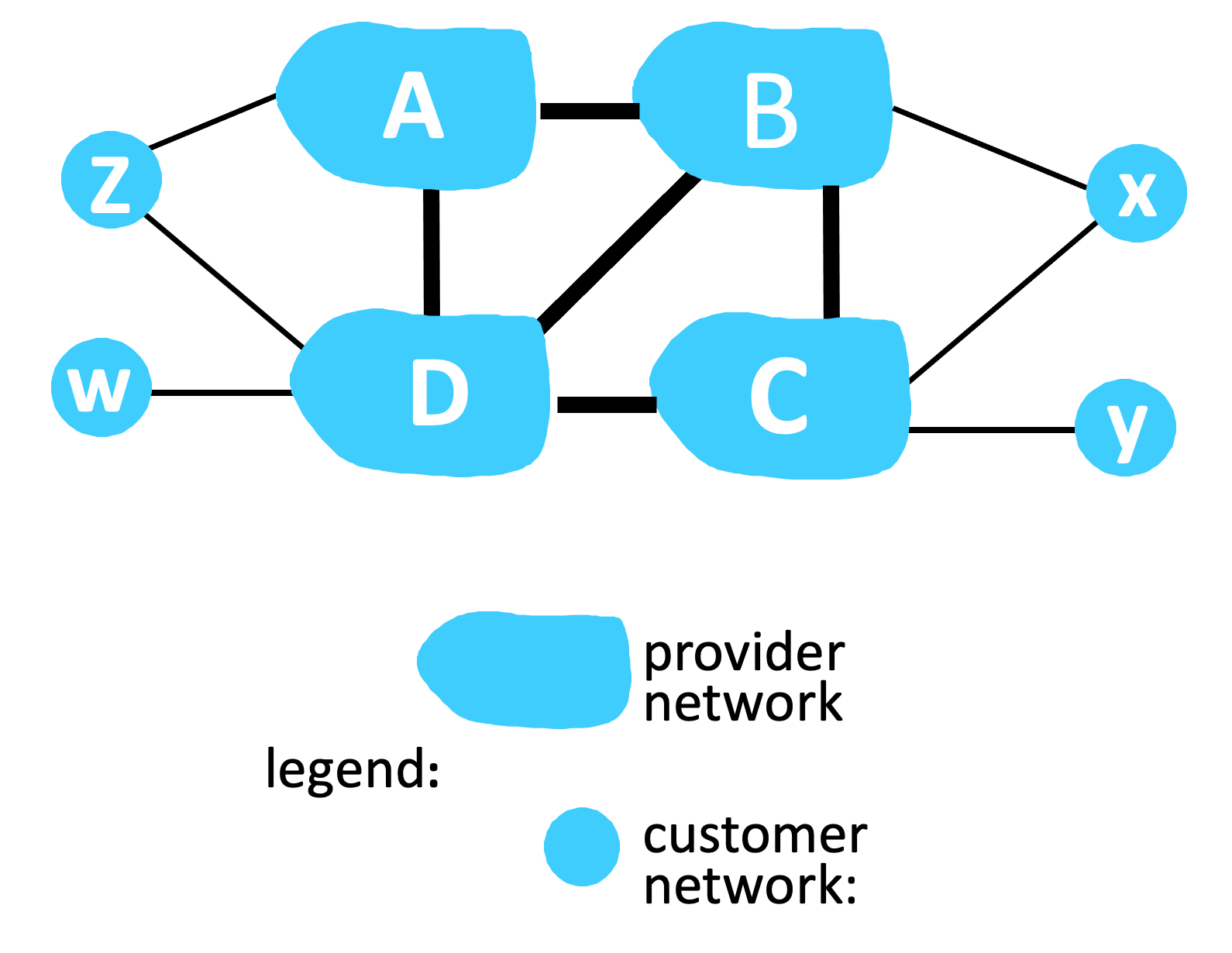5.02-3. Dijkstra’s Algorithm (2, part 3).  Consider the network shown below, and Dijkstra’s link-state algorithm. Here, we are interested in computing the least cost path from node y (note: not node u!) to all other nodes using Dijkstra’s algorithm. Using the algorithm statement used in the textbook and its visual representation, complete the first row in the table below showing the link state algorithm’s execution by matching the table entries (a), (b), (c), (d), and (e) with their values.  Write down your final [correct] answer, as you‘ll need it for the next question. [Note: You can find more examples of problems similar to this here.]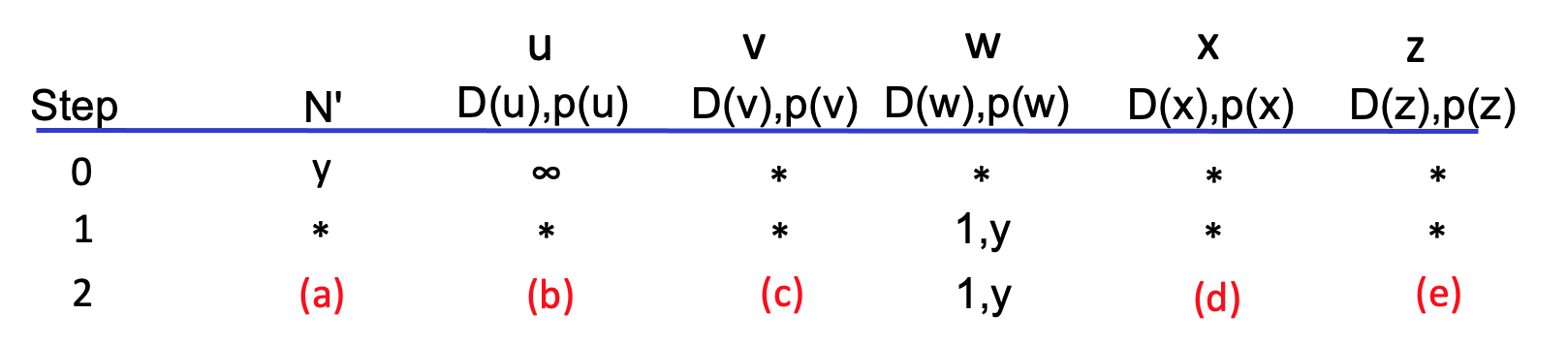–A.B.C.D.E.F.G.H.I.J. (a) –A.B.C.D.E.F.G.H.I.J. (b) –A.B.C.D.E.F.G.H.I.J. (c) –A.B.C.D.E.F.G.H.I.J. (d) –A.B.C.D.E.F.G.H.I.J. (e)
 A. 6,x B. ywv C. ywx D. 4,x E. 3,w F. 7,x G. 5,x H. 5,w I. ywz J. 9,w